## Solution to 1986 Problem 54

 The bound charge density is given by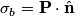\begin{align*}\sigma_b = \mathbf{P} \cdot \hat{\mathbf{n}}\end{align*}where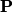$\mathbf{P}$ is the polarization vector in the dielectric and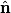$\hat{\mathbf{n}}$ is a normal vector that points from the dielectric to the conductor. The polarization vector in the dielectric is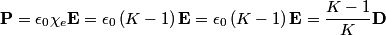\begin{align*}\mathbf{P} = \epsilon_0 \chi_e \mathbf{E} = \epsilon_0 \left(K - 1 \right) \mathbf{E} = \epsilon_0 \left(K - 1 ...The electric displacement produced by the charge density$\sigma$ is$\sigma$, and it is in the direction opposite$\hat{\mathbf{n}}$. Thus,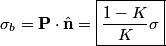\begin{align*}\sigma_b = \mathbf{P} \cdot \hat{\mathbf{n}} = \boxed{\frac{1 - K}{K} \sigma}\end{align*}Therefore, answer (E) is correct.